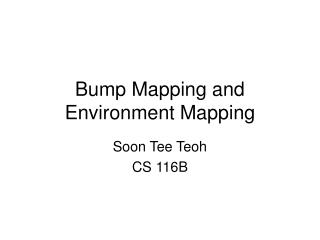DownloadDownload PresentationBump Mapping and Environment Mapping

# Bump Mapping and Environment Mapping

Download Presentation## Bump Mapping and Environment Mapping

- - - - - - - - - - - - - - - - - - - - - - - - - - - E N D - - - - - - - - - - - - - - - - - - - - - - - - - - -
##### Presentation Transcript

1. Bump Mapping and Environment Mapping Soon Tee Teoh CS 116B

2. Bump Mapping • Textures not effective for modeling rough surfaces, such as oranges and strawberries • Instead, we perturb the surface normals slightly. As normals are perturbed, so lighting is also slightly altered, giving the illusion of a rough surface.

3. Bump Mapping • Suppose that a surface is defined in parametric space (u,v). • Then, a bump map is a function b(u,v) such that for each u,v, b(u,v) represents the change in the height of the surface relative to the original flat surface. • The function b(u,v) can be specified by an analytic expression, or by a 2D table (matrix) of values. v u

4. Calculating the normal • Now, for each point on the surface, we need to calculate the new normal, given the bump map. • Let P(u,v) be a point on the surface. • Then, N = Pu x Pv is the normal at P(u,v). Let n be the unit vector of N. • where Pu and Pv represent the partial derivatives of P in the u and v directions respectively • Considering the bump map, updated surface position is P’(u,v) = P(u,v) + b(u,v)n • Approximate perturbed normal is N’ = N - bv(nxPu) + bu(nxPv) • where bu and bv represent the partial derivative of b in the u and v directions respectively • Therefore, given the bump map, we find N’ at each u,v location on the surface, and use n’ for lighting calculations.

5. Law of Reflection • Angle of reflection is equal and opposite to the incident angle, with respect to the surface normal. • Consequence of this law: If a point P is a distance d in front of the surface (measured in the direction of the surface normal), then its reflected point P’ appears to be the same distance d behind the surface. Normal N Reflected ray True ray True object Reflective surface Apparent ray Reflected object

6. Reflection • There are some common flat reflective surfaces which the viewer cannot go behind. • Examples of these are: • Flat water surface of a lake • Mirror against the wall • To fake reflection of a mirror against the wall, simply make a duplicate of the scene, but subjected to a reflection transformation so that all the duplicated objects are behind the mirror.

7. Environment Maps • Faking the reflection from a complex surface (such as a sphere) is more difficult. • One way to do it is using an environment map. • This method does not produce the correct reflection, but some approximation of it. Most humans are not able to tell the difference. • Environment Mapping: • Step 1: Use a wide angle projection from the center of the complex (e.g. spherical) object towards the camera position, and save the image. • Step 2: Use this image as a spherical texture map for the object. Use OpenGL’s automatic texture coordinate generation utilities.

8. Sphere Mapping Example // enable automatic texture coordinate generation glTexGeni(GL_S, GL_TEXTURE_GEN_MODE, GL_SPHERE_MAP); glTexGeni(GL_T, GL_TEXTURE_GEN_MODE, GL_SPHERE_MAP); glEnable(GL_TEXTURE_GEN_S); glEnable(GL_TEXTURE_GEN_T); // suppose that the texture has been loaded to d glBindTexture(GL_TEXTURE_2D, d); glPushMatrix(); glTranslatef(-1.2,-1.2,0.0); glRotatef(xrot,1.0,0.0,0.0); glRotatef(yrot,0.0,1.0,0.0); glRotatef(zrot,0.0,0.0,1.0); glutSolidTeapot(1); // draw a teapot with this sphere map glPopMatrix(); glPushMatrix(); glBindTexture(GL_TEXTURE_2D, TextureArray); glTranslatef(0.9,0.9,0.0); glutSolidSphere(1.0,20,20);// draw a sphere with this sphere map glPopMatrix(); glDisable(GL_TEXTURE_GEN_S); glDisable(GL_TEXTURE_GEN_T); We use this texture Sphere map to some 3D objects We get this result

9. Spherical Texture Map • The preceding example enables automatic texture coordinate generation. • It generates the s and t texture coordinates automatically. • GL_SPHERE_MAP is used as the method to generate the s and t texture coordinates. • The GL_SPHERE_MAP method generates the s and t texture coordinates based on the normal of the vertex. • This is good for using to simulate reflection, because reflection at any point is based on the normal at that point.

10. Cube Maps • Alternatively, we can use cube map for environment-mapping. • In the cube map method, instead of having a sphere surrounding a point, we have a cube surrounding a point. • A cube consists of six faces. Therefore, we take a picture of the environment from the point towards each of the six faces. • We then use this as the environment map. • The s and t texture coordinates to use for any vertex, like in the sphere map, are dependant on the normal of the vertex.

11. Automatic Texture Coordinate Generation • Automatic texture coordinate generation can be used for purposes other than spherical environment mapping. • They can simply be used to automatically generate the texture coordinates of an object. • Use GL_OBJECT_LINEAR instead of GL_SPHERE_MAP if you want to use the vertex coordinates rather than the vertex normals to generate texture coordinates.

12. Object coordinates example void sideGlutDisplay( void ) { float light_ambient = { 0.1, 0.1, 0.1, 1.0 }; // r, g, b, a float light_diffuse = { 1.0, 1.0, 1.0, 1.0 }; // r, g, b, a float light_position = { 0.0, 1.0, 1.0 , 0.0 }; // x, y, z, w glClearColor(0.0,0.0,0.0,0.0); glClear( GL_COLOR_BUFFER_BIT | GL_DEPTH_BUFFER_BIT ); glMatrixMode(GL_PROJECTION); glLoadIdentity(); glFrustum(-1.0,1.0,-(float)sideheight/(float)sidewidth,(float)sideheight/(float)sidewidth,1.0,100.0); glViewport(0,0,sidewidth,sideheight); glMatrixMode(GL_MODELVIEW); glLoadIdentity(); gluLookAt(5,0,5,0,0,0,0,1,0); glEnable(GL_LIGHTING); glEnable(GL_DEPTH_TEST); glLightfv(GL_LIGHT0, GL_AMBIENT, light_ambient); glLightfv(GL_LIGHT0, GL_DIFFUSE, light_diffuse); glLightfv(GL_LIGHT0, GL_POSITION, light_position); glEnable(GL_LIGHT0); glEnable(GL_TEXTURE_2D); JPEG_Texture(TextureArray, "marbleTexture.jpg", 0); glBindTexture(GL_TEXTURE_2D, TextureArray); glTexEnvi(GL_TEXTURE_ENV, GL_TEXTURE_ENV_MODE, GL_MODULATE); glTexParameteri(GL_TEXTURE_2D,GL_TEXTURE_WRAP_S,GL_REPEAT); glTexParameteri(GL_TEXTURE_2D,GL_TEXTURE_WRAP_T,GL_REPEAT); glTexGeni(GL_S, GL_TEXTURE_GEN_MODE, GL_OBJECT_LINEAR); glTexGeni(GL_T, GL_TEXTURE_GEN_MODE, GL_OBJECT_LINEAR); glEnable(GL_TEXTURE_GEN_S); glEnable(GL_TEXTURE_GEN_T); glutSolidTorus(1,2,20,20); glutSwapBuffers(); }